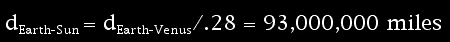# Why Is It Important?

Transits of Venus have long been useful to scientific research. In the 1700s, they helped us determine our distance to the Sun and gave us the first clue that Venus might have an atmosphere.

How do we know how far away the Sun is? Now we use radar to establish our distance from celestial objects, but before there was radar, we had to resort to trigonometry to figure out the astronomical unit (AU)—the distance between Earth and the Sun. In 1677, English astronomer Edmond Halley (of comet fame) proposed that a transit of Venus—and some geometry—could be used to determine the astronomical unit. Though Halley died in 1742, which was 19 years before the 1761 transit of Venus, other scientists followed his suggestion and journeyed to the ends of the Earth to make the necessary observations. The astronomical unit obtained from these observations, roughly 95 million miles, is respectably close to our current measure of 92,955,807.267 miles (149,597,870.691 kilometers).

It was also during the 1761 transit of Venus that observers noticed something strange: a fuzzy halo of light surrounding the dark spot of Venus. The fuzzy halo was only visible when Venus was at the Sun's edge. Scientists of the time concluded that Venus must have an atmosphere, and we've since confirmed that it does: a dense atmosphere of mainly carbon dioxide, with clouds of sulfuric acid.

Transits are still of interest to scientists today, because they can be used to find planets outside our solar system, also known as extrasolar planets, or exoplanets. Extrasolar planets are too distant and too dim to be viewed directly, but when one passes in front of (that is, transits) its parent star, it blocks a little bit of the star's light. (The dimming is detectable but extremely slight; just 1 percent for a giant planet like Jupiter and .01 percent for a planet the size of Earth.) Scientists analyzing the changing light from stars can not only identify the presence of a planet, but can also determine the planet's size, temperature, and atmospheric composition. The transit of Venus provides great practice for these scientists, allowing them to track the optical changes that take place during a transit.

In 2009, NASA launched the Kepler Mission, which uses a space telescope to search for Earth-sized exoplanets. Kepler watches more than 100,000 stars that are similar to our Sun, looking for variations in brightness caused by planets transiting their parent stars.

## Finding the AU: How the Transit of Venus Tells Us Our Distance from the Sun

By 1619, German astronomer Johannes Kepler had figured out the relative distances of all the planets from the Sun. For example, if the Earth's distance from the Sun is one astronomical unit (AU), then Venus's distance from the Sun is .72 AU, Mars's is 1.5 AU, and so on.

However, no one knew the value of AU, so the absolute distances between the celestial spheres were not known.

In 1716, English astronomer Edmond Halley proposed a method for calculating our distance from the Sun—the astronomical unit—using the transit of Venus.

The underlying principle behind Halley's method is something called parallax, the shift in position that comes from viewing an object from two different points.
What is parallax? Try this.

X

What Is Parallax?

Try this:
Point your index finger towards an object on the opposite side of the room. Look at it first with just your left eye, then switch to looking at it with just your right eye.

Can you see that the place across the room that your finger points to seems to jump, depending on which eye you use to look at it? That’s parallax . The farther away the object you point at, the more it seems to jump when you switch eyes.

Halley’s method uses parallax to find our distance from the Sun. Observers stationed far apart on Earth are the two eyes, Venus is the pointing finger, and the Sun is the object across the room.

Imagine two different people, one on each pole of the Earth, viewing the transit of Venus. The person on the North pole sees Venus following one path across the Sun. The person on the South pole sees Venus follow a slightly higher path, one that's shifted a little to the north.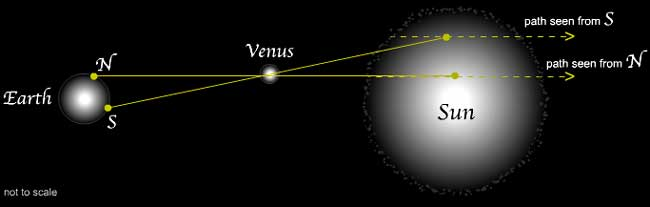Because we see the Sun as a circle, these two different paths will have different lengths. Halley proposed that an easy way to measure the difference between the lengths of these two paths would be to time the transits, using the four phases of the transit—the first, second, third, and fourth contacts—as indicators.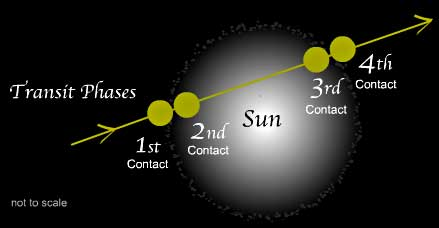With the two different paths known, the distance between the Earth and the Sun can be pretty easily calculated using trigonometry and Kepler's third law of planetary motion.

## Finding the AU: Calculation of the Sun-Earth Distance

During the transit of Venus, two observers on Earth view Venus at two different points on the Sun.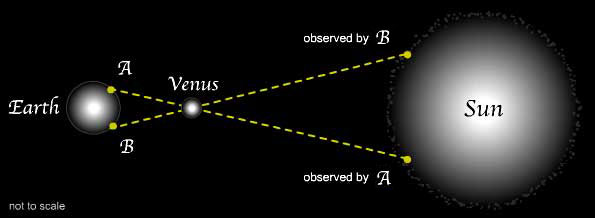We'll call the angle between the two paths measured from Earth E .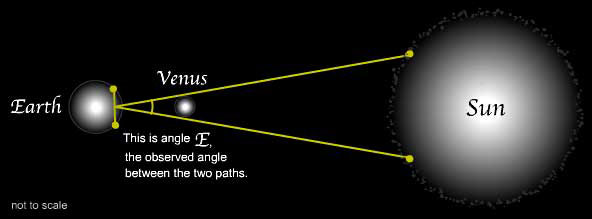Thanks to Kepler's third law , we know the relative distances of all the planets from the Sun. Case in point: We know that Venus's distance from the Sun is .72 times the Earth's distance from the Sun.

X

Kepler’s Third Law

Johannes Kepler’s third law of planetary motion established relative distances of all the planets from the Sun, but not absolute distances. Published in 1619, the law goes like this: The cube of a planet’s distance from the Sun is proportional to the square of its orbital period (one year for Earth, 5/8 of a year for Venus).

This distance relationship also tells us angle V , the angle between the two paths as seen from Venus: angle V is angle E divided by .72. (This is true only for small angles, which these are.)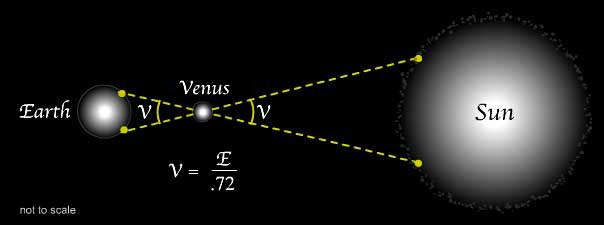## Finding the AU: Calculation of the Sun-Earth Distance

Besides the angle V , the only other thing we need to know is the distance between the two observers on Earth, at points A and B.

Call this distance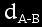.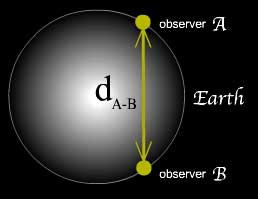Then, to get the distance from Venus to Earth, all we need is basic trigonometry. There is a right triangle formed between the Earth and Venus.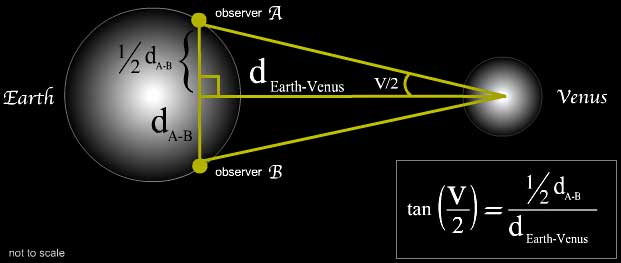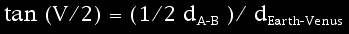Or, rearranging the equation: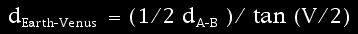For small angles, tan (1/2 A) = 1/2 tan A, so: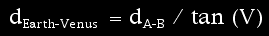Once we have the distance between Earth and Venus ,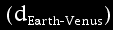it's easy to find the distance from the Earth to the Sun, again using Kepler's third law. This law tells us that the distance between the Earth and Venus is .28 times the distance between the Earth and the Sun.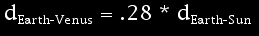So: